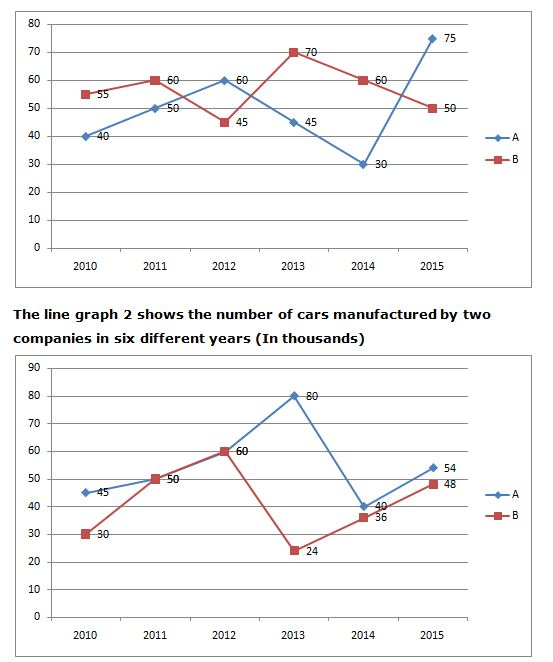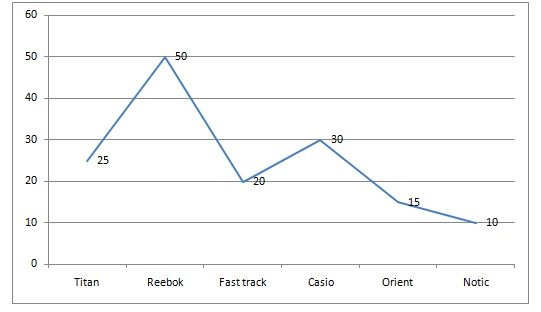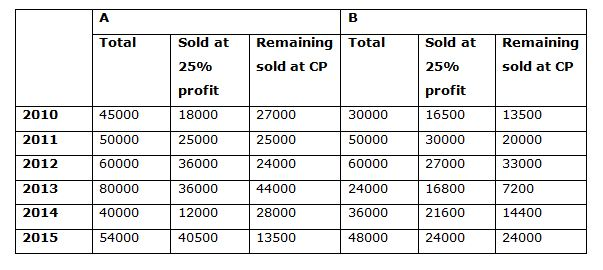# Quantitative Aptitude Questions (Data Interpretation) for SBI Clerk Mains 2018 Day-168

Dear Readers, SBI is conducting Online Examination for the recruitment of clerical cadre To enrich your preparation here we have providing new series of Data Interpretation – Quantitative Aptitude Questions. Candidates those who are appearing in SBI Clerk Mains Exams can practice these Quantitative Aptitude average questions daily and make your preparation effective.

[WpProQuiz 3124]

Click “Start Quiz” to attend these Questions and view Solutions

Directions (1-5): Study the following graph carefully and answer the given questions

The line graph shows the percentage of cars sold at 25% profit of two companies in six different years [remaining are sold at CP].1) What is the difference between the number of cars sold by company B at CP in the year 2012 and 2014 together and the no of cars sold by company A at 25% profit in the year 2010 and 2013 together?

a) 6000

b) 5400

c) 6600

d) 4800

e) 6400

2) What is the ratio between the no of cars sold by company A at 25% profit in the year 2011, 2012 and 2013 together and the no of cars sold by company B at CP in the year 2010, 2014 and 2015 together?

a) 219:134

b) 179:144

c) 412:319

d) 310:173

e) None of these

3) What is the difference between the total cars manufactured by company A and company B in the given years? (In thousands)

a) 81

b) 27

c) 54

d) 72

e) 80

4) No of cars manufactured by company B in the year 2012 and 2015 together is what percentage more/less than the no of cars manufactured by company A in the year 2010 and 2014 together?

a) 11

b) 13

c) 15

d) 27

e) 35

5) In which year, the total number of cars manufactured by company A and B together is maximum?

a) 2010

b) 2012

c) 2013

d) 2015

e) None of these

Directions (6-10): Study the following graph carefully and answer the following questions.

The following line graph shows that the percentage of profit when profit percentage is calculated on selling price of different types of watches sold by a shop owner.The table shows the cost price of different brand watches.

 Watches Cost price (in Rupees) Titan 4500 Reebok – Fast track 3200 Casio – Orient 2800 Notic –

6) If the total profit earned by selling Some Titan Watches is Rs 12000, then find the number of Titan watches to earn that profit.

a) 9

b) 6

c) 5

d) 8

e) 7

7) If Ratio of cost price of Orient watch and another Watch named Quartz is 7:9, then cost price of Quartz watch is approximately what percent more or less than cost price of Orient?

a) 87%

b) 25%

c) 29%

d) 30%

e) 65%

8) If the ratio of profit per watch of Fast track and Notic are in the ratio 4:5 then find the selling price of watch of the brand Notic?

a) Rs 50000

b) Rs 10000

c) Rs 70000

d) Rs 40000

e) Rs 60000

9) If the selling price of one Casio watch is 70% of the selling price one Fast track watch, then find the ratio of cost price of one Fast track watch to that of Casio watch.

a) 76:32

b) 55:43

c) 47:98

d) 78:74

e) 80:49

10) If the ratio of cost price of Reebok and Casio is 5:6 and the cost price of Reebok watch is 40% more than cost price of Orient Watch, then find the total selling price of Reebok watch and Casio watch together?

a) Rs.14560

b) Rs.6580

c) Rs.7840

d) Rs.4690

e) Rs.9870

Directions (1-5):Required difference = (18000+36000) – (33000+14400) = 54000 – 47400 = 6600

Required ratio = (25000+36000+36000): (13500+14400+24000)

= 97000: 51900 = 970: 519

Required difference = (45+50+60+80+40+54) – (30+50+60+24+36+48)

=>81 thousands

Required percentage = [(60+48)-(45+40)]/(45+40) *100

=>(108-85)/85 *100 = 23/85 *100 => 27.05%= 27% (Approximately)

In 2010 = 75

In 2011 = 100

In 2012 = 120

In 2013 = 104

In 2014 = 76

In 2015 = 102

Direction (6-10)

Given that Total profit= Rs 12000

Total selling price= 12000* 100/25=Rs 48000

Then Total cost price= 48000-12000= Rs 36000

Thus Number of Titan watches sold= 36000/4500=8

Cost price of Orient watch= Rs 2800

Then, Cost price of Quartz watch= 2800/7*9=Rs 3600

Required percentage= 800/2800*100= 29%

Let selling price of Fast track and Notic watches be Rs x and Rs y respectively.

Then, [(20% of x) / (10% of y)] = 4/5

x / y= 2/5

(i.e) 5x = 2y ………. (1)

For Fast track watch,

x= 3200+20x/100

x = 3200+x/5

x-x/5= 3200

4x/5= 3200

4x= 16000

x= 4000

Substituting this in (1), we get

y= Rs 10000

Selling price of Fast track watch= 3200*100/80=Rs 4000

Then selling price of Casio watch= 4000* 70/100= Rs 2800

Now cost price of one Casio watch= 2800*70/100=Rs 1960

Then Required ratio= 3200:1960= 80:49

Let selling price of Reebok be Rs x.

Cost price of Reebok= 2800* 140/100= Rs 3920

And Cost price of Casio= 3920/5*6= Rs 4704

Then Selling price of Reebok = 3920+50/100*x=x

x= Rs 7840

Also let y be the selling price of the Casio watch.

Then, 4704+ 30/100*y=y

47040+3y= 10y

7y= 47040

y=6720

Thus total selling price of Reebok and Casio watch= 7840+6720= Rs 14560

Daily Practice Test Schedule | Good Luck

 Topic Daily Publishing Time Daily News Papers & Editorials 8.00 AM Current Affairs Quiz 9.00 AM Quantitative Aptitude “20-20” 11.00 AM Vocabulary (Based on The Hindu) 12.00 PM General Awareness “20-20” 1.00 PM English Language “20-20” 2.00 PM Reasoning Puzzles & Seating 4.00 PM Daily Current Affairs Updates 5.00 PM Data Interpretation / Application Sums (Topic Wise) 6.00 PM Reasoning Ability “20-20” 7.00 PM English Language (New Pattern Questions) 8.00 PM